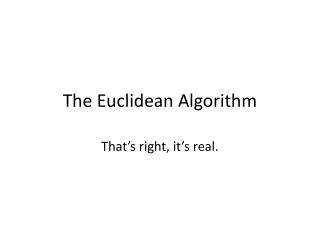DownloadDownload PresentationThe Euclidean Algorithm

# The Euclidean Algorithm

Download Presentation## The Euclidean Algorithm

- - - - - - - - - - - - - - - - - - - - - - - - - - - E N D - - - - - - - - - - - - - - - - - - - - - - - - - - -
##### Presentation Transcript

1. The Euclidean Algorithm That’s right, it’s real.

2. Duh it’s real. • One of the oldest known algorithms. • Used to find the greatest common divisor of two positive integers. • The greatest common divisor of a and b is expressed by gcd(a, b).

3. Mini Examples • For example: gcd(12, 18) = 6 • And: gcd(420, 66) = 6 • And: ...you get the idea…

4. In a Nut Shell Blue collar-ly, there’s a looping process going on that ends when the remainder is finally zero. At this point, the greatest common divisor is the last divisor used. This is the part where you do an example on the board, Harold.

5. How’s it work Take the larger of the two values that you are finding the gcd for. (I assume a>=b). Divide a by b, getting a quotient and a remainder. Next, divide b by the first remainder that you get, and thus a cycle has begun, and can be seen when arrows are drawn in.

6. And it can get CRAZY

7. Proving Euclid Wasn’t Nuts Buckle your seatbelts. This could get rough.

8. Euclidean Alg. Elsewhere • Proving two numbers are relatively prime. • Finding modular inverses to help solve and decrypt the RSA algorithm. (which I will NOT go over for your sanity) • RSA is public key cryptography. Ooooo look more examples!

9. Homework • Find the gcd(147, 105) • Find the gcd(Birthyear, Birthmonth^2) • Prove that 1193 and 317 are relatively prime. Then, try using Fermat’s Little Theorem to find the value of x in the equation: 317x = 1 mod 1193